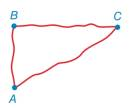Chapter 1.3, Problem 7E### Elementary Geometry for College St...

6th Edition
Daniel C. Alexander + 1 other
ISBN: 9781285195698

#### Solutions

Chapter
Section### Elementary Geometry for College St...

6th Edition
Daniel C. Alexander + 1 other
ISBN: 9781285195698
Textbook Problem
1 views

# In the figure 15-mile road from A to C is under construction. A detour from A to B of 5 miles and then from B to C of 13 miles must be taken. How much farther is the “detour’ from A to C than the road from A to C?Exercises 7, 8

To determine

To find:

The difference between the distances of the detour from A to C and the road from A to C.

Explanation

Given:

The given figure is,

The distance of road from A to C is 15 miles. A detour from A to B of 5 miles and then from B to C of 13 miles is taken.

Calculation:

Consider the given figure,

Figure (1)

Distance covered in detour from A to C is,

### Still sussing out bartleby?

Check out a sample textbook solution.

See a sample solution

#### The Solution to Your Study Problems

Bartleby provides explanations to thousands of textbook problems written by our experts, many with advanced degrees!

Get Started

#### Find the number n such that i=1ni=78.

Single Variable Calculus: Early Transcendentals

#### 5. Find the sum of the first 60 terms of the arithmetic sequence

Mathematical Applications for the Management, Life, and Social Sciences

#### In Exercises 914, evaluate the expression. 13. (323)(435)293

Applied Calculus for the Managerial, Life, and Social Sciences: A Brief Approach

#### The unit vector in the direction of is:

Study Guide for Stewart's Multivariable Calculus, 8th